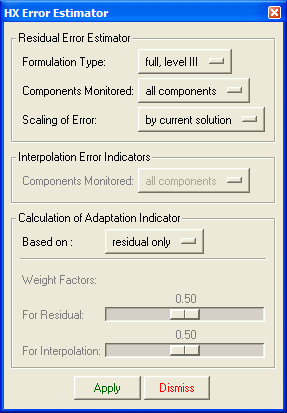﻿ Error Estimation Parameters
Manufacturing Solutions

# Error Estimation Parameters# Error Estimation ParametersThe Error Estimator option on the Adaptation menu allows you to select between two versions of residual estimates, an inexpensive version and an accurate version. Alternatively, interpolation type error indicators can be calculated. Indicators used to adapt the mesh are also chosen on this menu as a combination of the residual estimates and interpolation indicators. HyperXtrude uses both residual based and interpolation based error estimates.The Error Estimator panel

The Error Estimator panel provides the following options:

1) For residual error estimator:

Description

### Formulation Type

Select the version of the residual estimate to be used. Full Level II is the most expensive one as it uses the interior bubble functions to estimate the errors. Full Level I is the same as Full Level II except it ignores the interior bubble function. Full Level I produces inaccurate/unreliable values for non-Newtonian fluid flow problems

### Components Monitored

Equation Set is the set of governing equations to be examined by the residual error estimator. Valid options are All Equations, Momentum Only and Energy Only.

### Scaling of Error

This a scale factor to use on residual error estimates. Currently the only active option is By current solution. This option scales each estimate by dividing it by the mesh-wise norm of the current solution. The resulting estimates are non-dimensionalized and can be interpreted as a percentage of the solution. For example, if an element has a scaled estimate of 0.03 and the whole mesh has a scaled estimate of 0.05, this indicates the solution is on the average in error of 5 percent  according to the particular norm. Furthermore, 20 percent  of the error occurs in that particular element. This percentage is calculated according to (0.05 - (0.05*0.05 - 0.03*0.03)^(0.5))/0.05).

2) For error indicator:

Description

### Components Monitored

Select the number of solution components monitored in calculating the interpolation error indicators.

3) For both (estimator and indicator):

Description

### Based On

This option lets you select which combination of residual estimates and interpolation indicators to use when adapting the mesh. The options available are Residual Only, Interpolation Only, Maximum of Both, and Weighted Sum of Both. If the Weighted Sum of Both option is selected, the Weight Factors portion of the Error Estimator panel becomes active. On this portion of the panel you can choose weight factors by which to multiply the residual and interpolation errors to obtain a weighted sum.